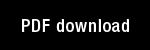Learning and energy-entropy dependence in some nonlinear functional-differential systems

Author(s): Grossberg, S. |

Year: 1969

Citation: Bulletin of the American Mathematical Society, 75, 1238-1242

Abstract: 1. Introduction. This note describes limiting and oscillatory fea-tures of some nonlinear functional-differential systems having appli-cations in learning and nonstationary prediction theory. The mainresults discuss systems of the form
(1) z,(t) = A(W,, t)xt(t) + E Bk(W,, t)zkti(t) + C.(t)kEJand(2) i i i (t) = Di(Wg, t)zi:(t) + Ej(W,, t)x:(t),where i E I, j E J, and I and J are finite sets of indices such that eitherI = J or It'1 J = J. The coefficients are continuous functions of t,dependent perhaps on the 111 (1 + I JI) dimensional vector functionW=(x;,z;;: iEI, jEJ) evaluated at times no later than t, and onknown functions of t. All coefficients B; and E; are also nonnegative,and the initial data and inputs C{ are nonnegative and continuous.The main results discuss the probabilities y;; (t) =z;;(t) [ Ekâ‚¬rz;k(t) ]-'and Xi(t) =x;(t) [ Ekerxk(t) ]-1 defined for iEI and jEJ, given choicesof initial data and coefficient functionals for which (1) and (2) has aunique bounded solution.These results apply for example to systems of the form [. . .]

Topics: Mathematical Foundations of Neural Networks, Models: Other,